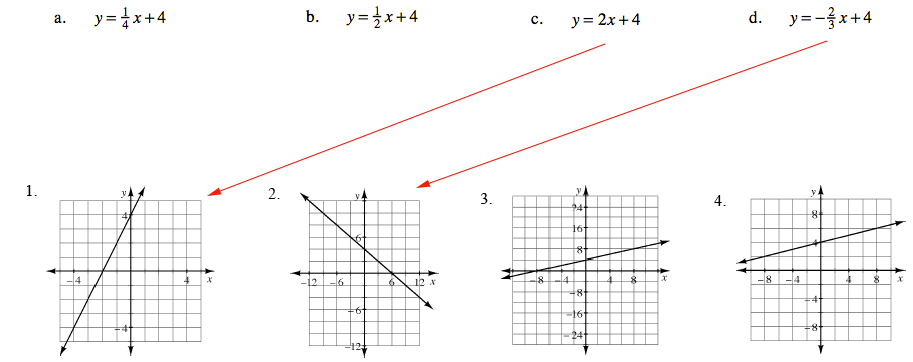### Home > CCA > Chapter Ch2 > Lesson 2.2.3 > Problem2-74

2-74.

MATCH-A-GRAPH

Match the following graphs with their equations. Pay special attention to the scaling of each set of axes. Explain how you found each match. Homework Help ✎

 a. $y=\frac{1}{4}x+4$ b. $y=\frac{1}{2}x+4$ c. $y=2x+4$ d. $y=−\frac{2}{3}x+4$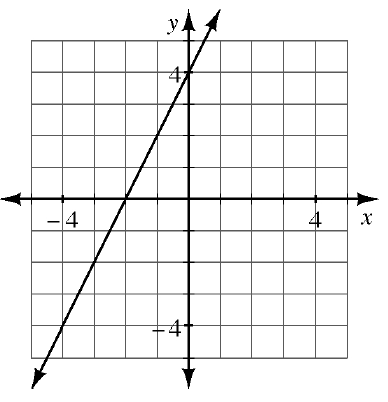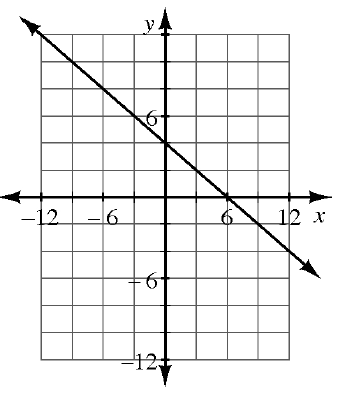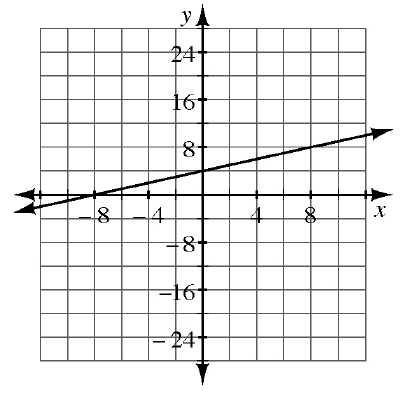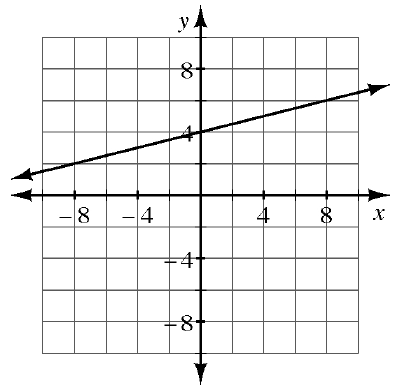Find the corresponding graph for each equation by starting with the $y$-intercept, then use the slope to find the next point.